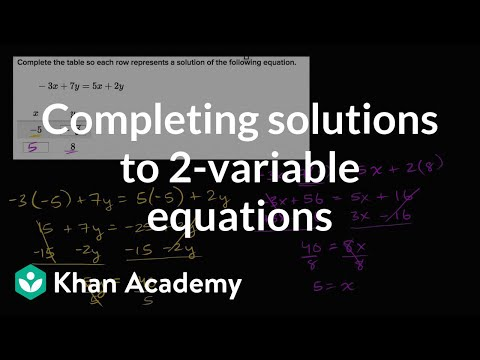# Systems of equations with elimination: x - 4y = -18 and -x + 3y = 11 (Full video)

Description: Sal demonstrates how to solve the system of equations x - 4y = -18 and -x + 3y = 11 using the elimination method. This first equation, X minus four Y is equal to negative 18, and the second equation, negative X plus three Y is equal to 11. And so if you find the X and Y pair that satisfy both, that would be the intersection of the lines, so let's do that. So actually, I'm just gonna rewrite the first equation over here, so I'm gonna write X minus four Y is equal to negative 18.

### Other videos you might be interested in### Solving Systems of Equations by Substitution (Full video)

#### Student Achievement Partners